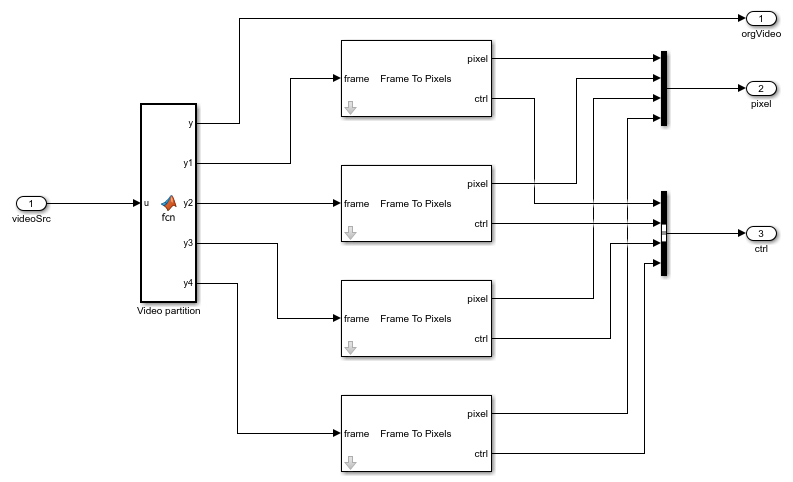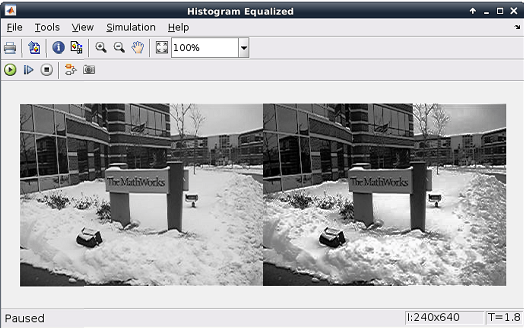# Histogram Equalization

This example shows how to use the Vision HDL Toolbox™ Histogram library block to implement histogram equalization.

This example model provides a hardware-compatible algorithm. You can generate HDL code from this algorithm, and implement it on a board using a Xilinx™ Zynq™ reference design. See Histogram Equalization with Zynq-Based Hardware (Vision HDL Toolbox Support Package for Xilinx Zynq-Based Hardware).

### Introduction

The model shows how to use the Histogram library block to enhance the contrast of images by applying the histogram equalization. To learn more, refer to the Histogram block reference page. There are three components in this histogram equalization example.

• Video Partition partitions a big image into four non-overlapping small images for parallel histogram computation.

• HDLHistogram computes the accumulated histogram of the image.

• Equalization applies the equalized histogram to the original image and generates the contrast-enhanced image.### Video Partition

There are use cases where histogram is computed over an entire image, or over small regions-of-interest representing sections of the image. Computing histogram of a big image is time consuming. The video partition component in this example divides a big image into four non-overlapping small images. Histogram is computed over the four small images simultaneously. Each input frame is partitioned into four 120 by 160 small images. Each small image is connected to a Frame To Pixels block to generate pixel streams and corresponding control signals.### HDLHistogram

HDLHistogram subsystem is optimized for HDL code generation. The histogram of the pixel streams is computed using the Histogram block. Because the input image is grey scale with data type uint8, the input pixels are grouped into 256 bins. The model reads the calculated histogram bins sequentially once the block asserts the readRdy signal. The bin values are sent for cumulative histogram calculation. After all 256 bin values are read, the model asserts binReset to reset all bins to zero. The collected histogram of each small image is then added together to compute the accumulated histogram of the big image.The timing diagram of reading and resetting the histogram bins is shown in the following figure.### Equalization

Histogram equalization can be applied to the current frame where the accumulated histogram was calculated, or the frame after. If applying to the current frame, the input video needs to be stored. This example delays the input video by one frame and performs uniform equalization to the original video. The equalized video is then compared with the original video.### HDL Code Generation

The HDL code generated from the Histogram was synthesized using Xilinx ISE on a Virtex6 (XC6VLX240T-1FFG1156) FPGA, and the circuit ran at about 190 MHz, which is sufficient to process the data in real time.

To check and generate HDL code of this example, you must have an HDL Coder™ license.

You can use the commands

```makehdl('HistogramEqualizationHDL/HDLHistogram') ```

or

```makehdltb('HistogramEqualizationHDL/HDLHistogram') ```

to generate HDL code and test bench for the HDLHistogram subsystem. Note: Test bench generation takes a long time due to the large data size. Consider reducing the simulation time before generating the test bench.

## SupportGet trial now